### Home > CALC > Chapter 3 > Lesson 3.3.4 > Problem3-140

3-140.
1. Evaluate each limit. If the limit does not exist, say so but also state if y is approaching positive or negative infinity. Homework Help ✎

1.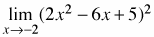2.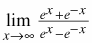3.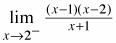4.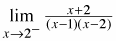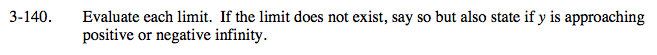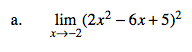Evaluate.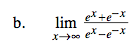As in all limits in which x→∞ or x→−∞, compare only the highest power in the numerator and denominator.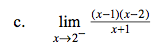Careful! This one can be deceptive. Just because this is a one-sided limit, does not mean that the limit→∞ or −∞. That will only be the case if there is a zero in the denominator that cannot be cancelled out.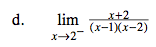This limit is asking you to determine whether the function approaches its vertical asymptote at x = 2 in a positive or negative direction when looked at from the left.

Choose a value a little to the left of 2 and evaluate. You only need to determine if the limit is positive or negative.

$\lim_{x\rightarrow 1.9}\frac{1.9+2}{(1.9-1)(1.9-2)}=\lim_{x\rightarrow 1.9}\frac{+}{(+)(-)}$

= negative value

Limit does not exist, but y→−∞.